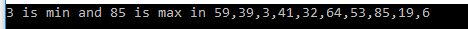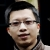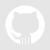C# 7 中的 Tuple 特性已翻译 100%

oschina 投递于 2017/03/16 14:41 (共 6 段, 翻译完成于 03-17)

参与翻译 (1人) : 承蒙

Tuple是异类对象的有序序列。 我们经常可以写出返回多个值的方法，所以我们需要创建一个包含多个数据元素的简单结构。 为了支持这些情况，Tuple 被添加到 C＃。 Tuple 是包含多个字段用来表示数据成员的轻量级数据结构。

using System.Collections.Generic;

namespace TupleApp
{
class Operation
{
internal int FindMinMax(List<int> list, out int min)
{
int maximum = int.MinValue, minimum = int.MaxValue;
list.ForEach(n =>
{
minimum = n < minimum ? n : minimum;
maximum = n > maximum ? n : maximum;
});
min = minimum;
return maximum;
}
}
}

using System;
using System.Collections.Generic;
using System.Linq;
using static System.Console;

namespace TupleApp
{
class Program
{
static void Main(string[] args)
{
List<int> numbers = Enumerable.Range(1, 100).OrderBy(x => Guid.NewGuid()).Take(10).ToList();
int minimum = 0, maximum = 0;
Operation operation = new Operation();
maximum = operation.FindMinMax(numbers, out minimum);
Write(\$"{minimum} is min and {maximum} is max in {String.Join(",", numbers)}");
}
}
}namespace TupleApp
{
struct CalculateData
{
public int Minimum { get; set; }
public int Maximum { get; set; }
}
}

using System.Collections.Generic;

namespace TupleApp
{
class Operation
{
internal CalculateData FindMinMax(List<int> list)
{
int maximum = int.MinValue, minimum = int.MaxValue;
list.ForEach(n =>
{
minimum = n < minimum ? n : minimum;
maximum = n > maximum ? n : maximum;
});
CalculateData data = new CalculateData
{
Minimum = minimum,
Maximum = maximum
};
return data;
}
}
}

using System;
using System.Collections.Generic;
using System.Linq;
using static System.Console;

namespace TupleApp
{
class Program
{
static void Main(string[] args)
{
List<int> numbers = Enumerable.Range(1, 100).OrderBy(x => Guid.NewGuid()).Take(10).ToList();
Operation operation = new Operation();
CalculateData data = operation.FindMinMax(numbers);
Write(\$"{data.Minimum} is min and {data.Maximum} is max in {String.Join(",", numbers)}");
}
}
}C＃7 引入了定义元组的新方法。 Tuple 是从方法返回多个值的另一个选择。 它可以保存多个不同类型的值。 要在应用程序中使用 Tuple，我们需要安装 System.ValueTuple NuGet 包.using System.Collections.Generic;

namespace TupleApp
{
class Operation
{
internal (int, int) FindMinMax(List<int> list)
{
int maximum = int.MinValue, minimum = int.MaxValue;
list.ForEach(n =>
{
minimum = n < minimum ? n : minimum;
maximum = n > maximum ? n : maximum;
});
return (minimum, maximum);
}
}
}

using System;
using System.Collections.Generic;
using System.Linq;
using static System.Console;

namespace TupleApp
{
class Program
{
static void Main(string[] args)
{
List<int> numbers = Enumerable.Range(1, 100).OrderBy(x => Guid.NewGuid()).Take(10).ToList();
Operation operation = new Operation();
(int, int) data = operation.FindMinMax(numbers);
Write(\$"{data.Item1} is min and {data.Item2} is max from {String.Join(",", numbers)}");
}
}
}using System.Collections.Generic;

namespace TupleApp
{
class Operation
{
internal (int Minimum, int Maximum) FindMinMax(List<int> list)
{
int maximum = int.MinValue, minimum = int.MaxValue;
list.ForEach(n =>
{
minimum = n < minimum ? n : minimum;
maximum = n > maximum ? n : maximum;
});
return (minimum, maximum);
}
}
}

using System;
using System.Collections.Generic;
using System.Linq;
using static System.Console;

namespace TupleApp
{
class Program
{
static void Main(string[] args)
{
List<int> numbers = Enumerable.Range(1, 100).OrderBy(x => Guid.NewGuid()).Take(10).ToList();
Operation operation = new Operation();
var data = operation.FindMinMax(numbers);
Write(\$"{data.Minimum} is min and {data.Maximum} is max from {String.Join(",", numbers)}");
}
}
}Tuple 是异类对象的有序序列。 当一个方法需要返回多个值的时候使用它。Tuple 实例的条目数是固定的。Tuple 有最大数目为 8 项的限制。 如果我们想创建一个带有更多项的 Tuple，我们必须创建嵌套的 Tuple。 Tuple 的第八项必须是另一个 Tuple。

评论(31)

l

y

引用来自“小杰瑞”的评论

MS在C# 语言上面下了不少功夫，golang的多返回值也借鉴来了...我只想说，语言只不过是你想表达逻辑的一种方式，最重要的是不管用什么工具，最后生产出来的软件产品是不是更有价值。
tuple这玩意儿上世纪就有了，又不是贵go发明的。而且go里用tuple代替exception根本是一种反模式
f引用来自“eechan”的评论引用来自“冬天之雪”的评论

Tuple虽然早就有，但是一直不好用。刚随 C# 7 发布出来的语义化 Tuple 和解构才真正可能让 Tuple 好用起来。以前返回多值用 Tuple 的 Item1、Item2 …… 脑壳痛，用匿名对象+dynamic又会损失静态检查，所以 C#7 这个 Tuple 满好的。C#优雅得不容质疑，不过我在等linux的.net快点完善